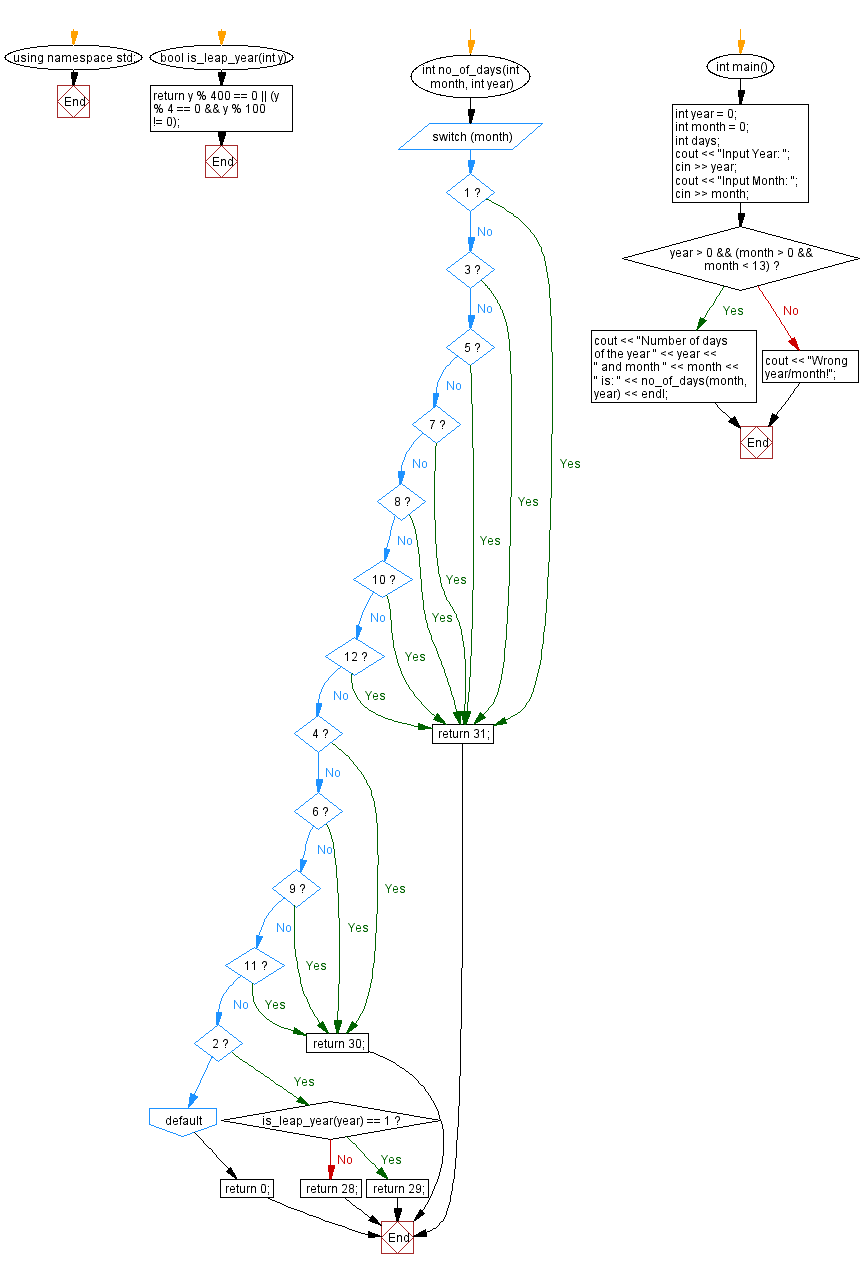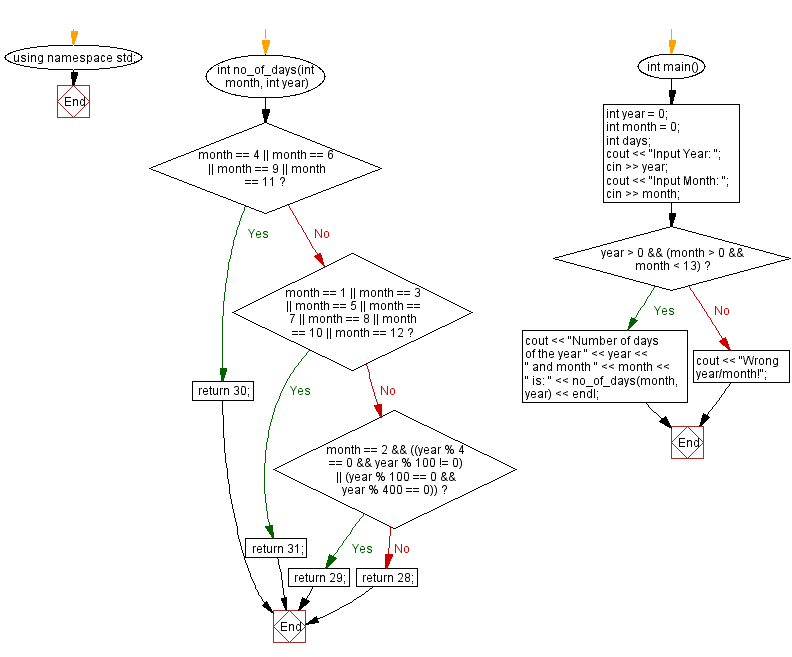﻿ C++ : Number of days of a month from a given year and month

# C++ Exercises: Number of days of a month from a given year and month

## C++ Date: Exercise-5 with Solution

Write a C++ program to compute the number of days in a month for a given year and month.

Sample Solution-1:

C++ Code:

``````#include <iostream>

using namespace std;
bool is_leap_year(int y) {
return y % 400 == 0 || (y % 4 == 0 && y % 100 != 0);
}
int no_of_days(int month, int year) {
switch (month) {
case 1:
case 3:
case 5:
case 7:
case 8:
case 10:
case 12:
return 31;
break;

case 4:
case 6:
case 9:
case 11:
return 30;
break;

case 2:
if (is_leap_year(year) == 1)
return 29;
else
return 28;
break;
default:
break;
}

return 0;
}

int main() {
int year = 0;
int month = 0;
int days;
cout << "Input Year: ";
cin >> year;
cout << "Input Month: ";
cin >> month;
if (year > 0 && (month > 0 && month < 13)) {
cout << "Number of days of the year " << year << " and month " << month << " is: " << no_of_days(month, year) << endl;

} else
cout << "Wrong year/month!";
}
``````

Sample Output:

```Input Year: 2019
Input Month: 04
Number of days of the year 2019 and month 4 is: 30
```

Flowchart:Sample Solution-2:

C++ Code:

``````#include <iostream>

using namespace std;

int no_of_days(int month, int year)
{

if (month == 4 || month == 6 || month == 9 || month == 11)
{
return 30;
}
else if (month == 1 || month == 3 || month == 5 || month == 7 || month == 8 || month == 10 || month == 12)
{
return 31;
}
else if (month == 2 && ((year % 4 == 0 && year % 100 != 0) || (year % 100 == 0 && year % 400 == 0)))
{
return 29;
}
else
{
return 28;
}
}

int main()
{
int year = 0;
int month = 0;
int days;
cout << "Input Year: ";
cin >> year;
cout << "Input Month: ";
cin >> month;
if (year > 0 && (month > 0 && month < 13))
{
cout << "Number of days of the year " << year << " and month " << month << " is: " << no_of_days(month, year) << endl;

} else
cout << "Wrong year/month!";
}
``````

Sample Output:

```Input Year: 2019
Input Month: 04
Number of days of the year 2019 and month 4 is: 30
```

Flowchart:C++ Code Editor: